# 10k NTC Thermistor Thermal Resistor Temperature Sensor NTC-MF52AT

## 10k NTC Thermistor Thermal Resistor Temperature Sensor NTC-MF52AT

SKU:b844,IMP2000,Th1000,A

Regular price Rs.10
Regular price Sale price Rs.10
Sale Sold out
Shipping calculated at checkout.

The MF52 thermistor is a small-sized,epoxy-resin coated NTC resistor made from new-type material with new craftsmanship. It is featured with advantages including high precision and quick reaction.Features:
Lacquer-coated thermistor disk

Specification:
Thermistor Type: NTC Thermistor
Resistance: 1K ohm
Resistance Tolerance: ±5%
B Constant: 3950K
B Constant Tolerance: ±1%
Temperature Range: -40 to +125 degree

Package Included:

• 1pc 10k OHM NTC MF52AT 3950 Thermistor Resistor NTC-MF52AT MF52 10K + -1% Thermal Resistor.
•

NTC is the abbreviation of Negative Temperature Coefficient, which means negative temperature coefficient. It generally refers to semiconductor materials or components with a large negative temperature coefficient. The so-called NTC thermistor is a negative temperature coefficient thermistor. It is made of metal oxides such as manganese, cobalt, nickel and copper as the main materials and made by ceramic technology. These metal oxide materials all have semiconductor properties because they are completely similar to semiconductor materials such as germanium and silicon in terms of conduction. When the temperature is low, the number of carriers (electrons and holes) of these oxide materials is small, so the resistance value is higher; as the temperature rises, the number of carriers increases, so the resistance value decreases. The NTC thermistor varies from 100 to 1,000,000 ohms at room temperature, and the temperature coefficient is -2% to -6.5%. NTC thermistor can be widely used in temperature measurement, temperature control, temperature compensation and other aspects.

NTC negative temperature coefficient thermistor composition

NTC (Negative Temperature Coefficient) refers to the thermistor phenomenon and material whose resistance decreases exponentially with temperature rise and has a negative temperature coefficient. The material is a semiconductor ceramic made of two or more metal oxides such as manganese, copper, silicon, cobalt, iron, nickel, zinc, etc., which are fully mixed, formed, and sintered, and can be made into a negative temperature coefficient (NTC) thermistor. The resistivity and material constants vary with the material composition ratio, sintering atmosphere, sintering temperature and structural state. Non-oxide NTC thermistor materials represented by silicon carbide, tin selenide, and tantalum nitride have also appeared.

NTC thermosensitive semiconducting ceramics are mostly oxide ceramics with spinel structure or other structures, which have a negative temperature coefficient, and the resistance value can be approximately expressed as:

Where RT and RT0 are the resistance values ​​at temperature T and T0 respectively, and Bn is the material constant. The resistivity of the ceramic grain itself changes due to temperature changes, which is determined by the characteristics of the semiconductor.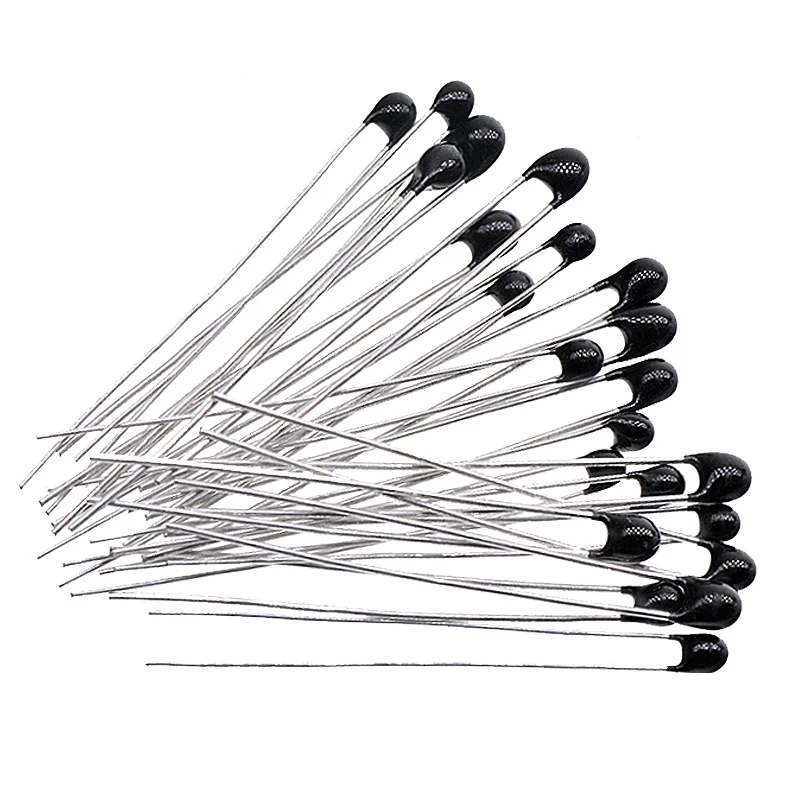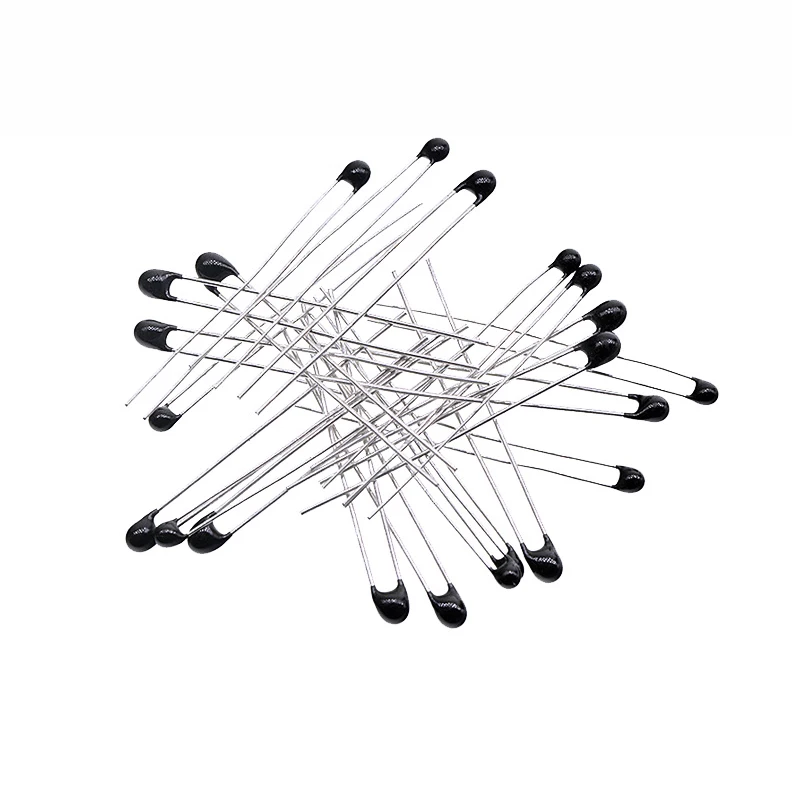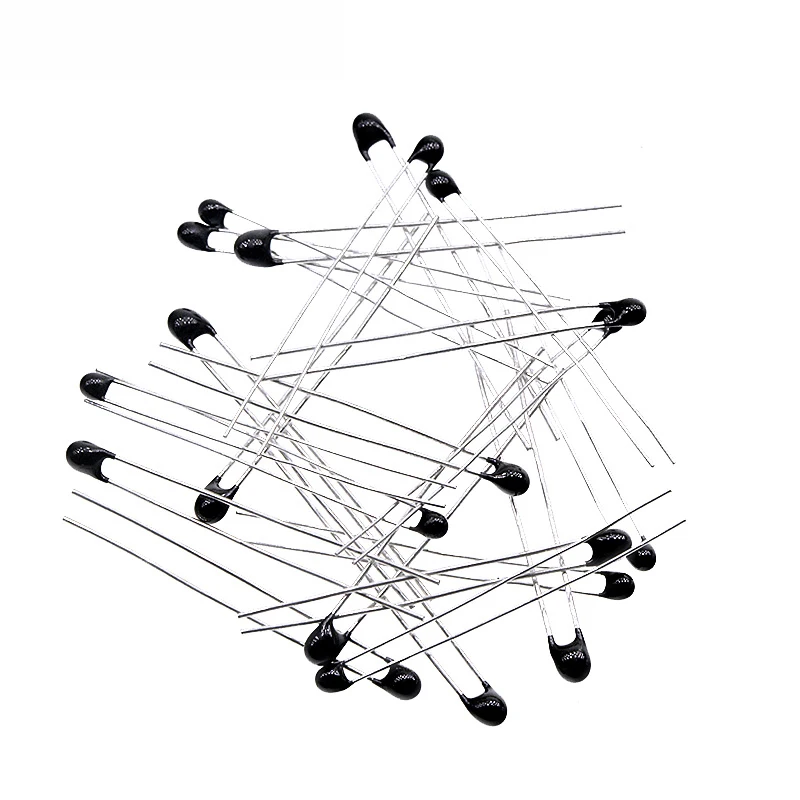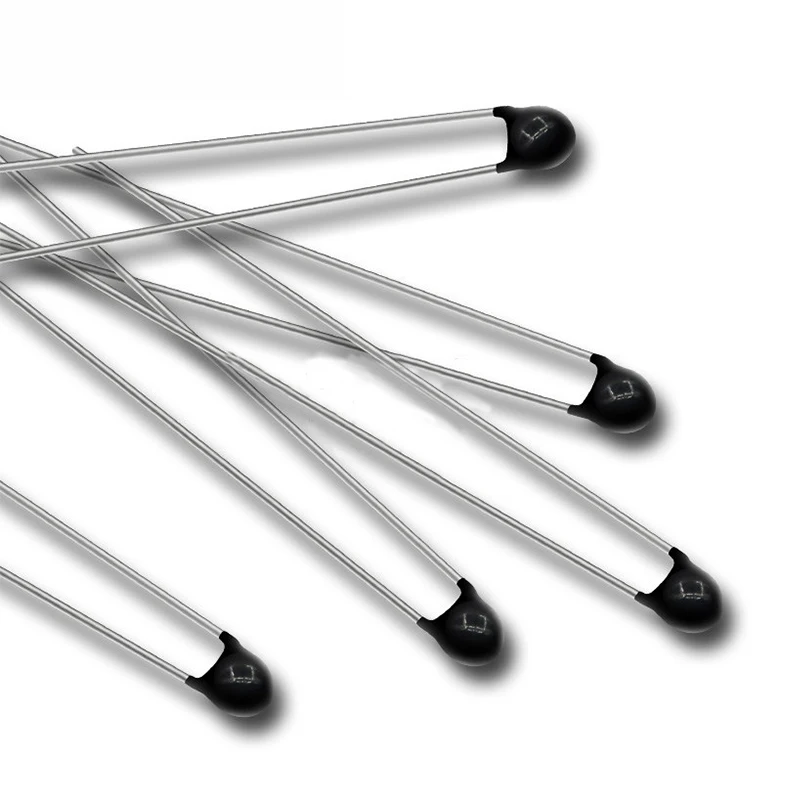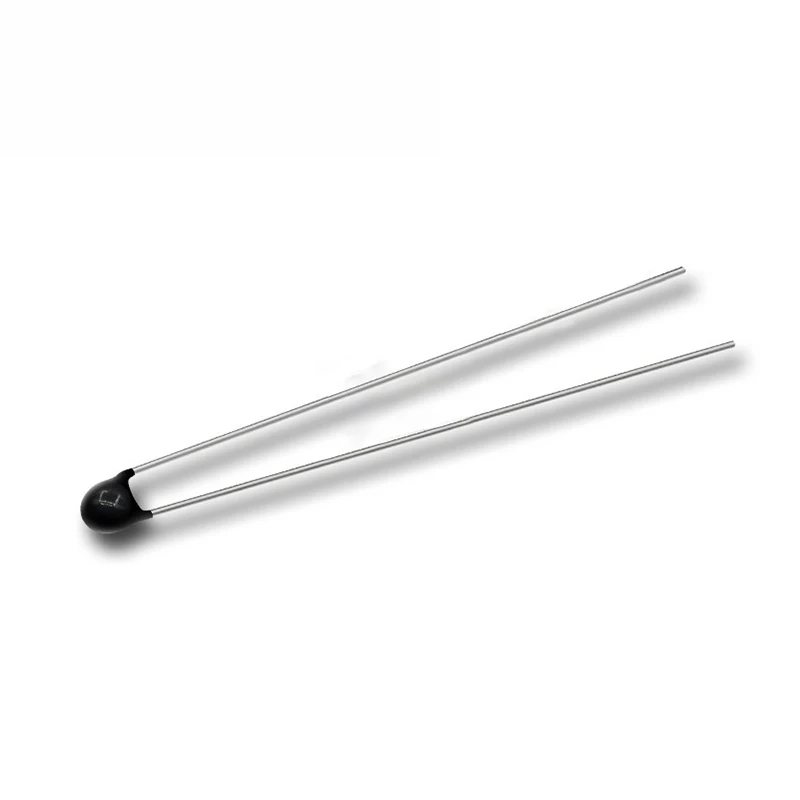View full details

## Description

The MF52 thermistor is a small-sized,epoxy-resin coated NTC resistor made from new-type material with new craftsmanship. It is featured with advantages including high precision and quick reaction.Features:
Lacquer-coated thermistor disk

Specification:
Thermistor Type: NTC Thermistor
Resistance: 1K ohm
Resistance Tolerance: ±5%
B Constant: 3950K
B Constant Tolerance: ±1%
Temperature Range: -40 to +125 degree

Package Included:

• 1pc 10k OHM NTC MF52AT 3950 Thermistor Resistor NTC-MF52AT MF52 10K + -1% Thermal Resistor.
•

NTC is the abbreviation of Negative Temperature Coefficient, which means negative temperature coefficient. It generally refers to semiconductor materials or components with a large negative temperature coefficient. The so-called NTC thermistor is a negative temperature coefficient thermistor. It is made of metal oxides such as manganese, cobalt, nickel and copper as the main materials and made by ceramic technology. These metal oxide materials all have semiconductor properties because they are completely similar to semiconductor materials such as germanium and silicon in terms of conduction. When the temperature is low, the number of carriers (electrons and holes) of these oxide materials is small, so the resistance value is higher; as the temperature rises, the number of carriers increases, so the resistance value decreases. The NTC thermistor varies from 100 to 1,000,000 ohms at room temperature, and the temperature coefficient is -2% to -6.5%. NTC thermistor can be widely used in temperature measurement, temperature control, temperature compensation and other aspects.

NTC negative temperature coefficient thermistor composition

NTC (Negative Temperature Coefficient) refers to the thermistor phenomenon and material whose resistance decreases exponentially with temperature rise and has a negative temperature coefficient. The material is a semiconductor ceramic made of two or more metal oxides such as manganese, copper, silicon, cobalt, iron, nickel, zinc, etc., which are fully mixed, formed, and sintered, and can be made into a negative temperature coefficient (NTC) thermistor. The resistivity and material constants vary with the material composition ratio, sintering atmosphere, sintering temperature and structural state. Non-oxide NTC thermistor materials represented by silicon carbide, tin selenide, and tantalum nitride have also appeared.

NTC thermosensitive semiconducting ceramics are mostly oxide ceramics with spinel structure or other structures, which have a negative temperature coefficient, and the resistance value can be approximately expressed as:

Where RT and RT0 are the resistance values ​​at temperature T and T0 respectively, and Bn is the material constant. The resistivity of the ceramic grain itself changes due to temperature changes, which is determined by the characteristics of the semiconductor.## Customer Reviews

Based on 1 review
100%
(1)
0%
(0)
0%
(0)
0%
(0)
0%
(0)
A